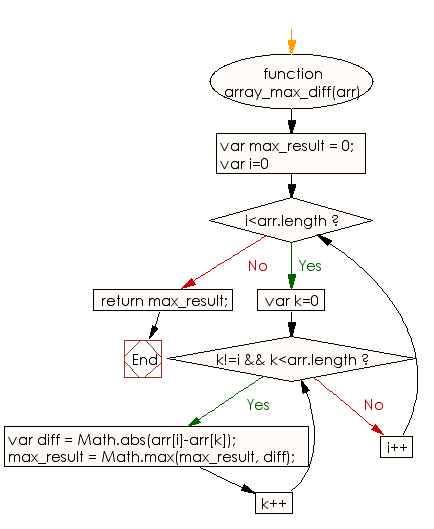# JavaScript: Find the maximum difference among all possible pairs of a given array of integers

## JavaScript Basic: Exercise-93 with Solution

Write a JavaScript program to find the maximum difference among all possible pairs of a given array of integers.

Sample Solution:

HTML Code:

``````<!DOCTYPE html>
<html>
<meta charset="utf-8">
<meta name="viewport" content="width=device-width">
<title>Find the maximum difference among all possible pairs of a given array of integers.</title>
<body>

</body>
</html>
```
```

JavaScript Code:

``````function array_max_diff(arr) {

var max_result = 0;

for(var i=0;i<arr.length;i++)
{
for(var k=0; k!=i && k<arr.length; k++)
{
var diff = Math.abs(arr[i]-arr[k]);
max_result = Math.max(max_result, diff);
}
}
return max_result;
}
console.log(array_max_diff([1, 2, 3, 8, 9]))
console.log(array_max_diff([1, 2, 3, 18, 9]))
console.log(array_max_diff([13, 2, 3, 8, 9]))
```
```

Sample Output:

```8
17
11
```

Flowchart:ES6 Version:

``````function array_max_diff(arr) {

let max_result = 0;

for(let i=0;i<arr.length;i++)
{
for(let k=0; k!=i && k<arr.length; k++)
{
const diff = Math.abs(arr[i]-arr[k]);
max_result = Math.max(max_result, diff);
}
}
return max_result;
}
console.log(array_max_diff([1, 2, 3, 8, 9]))
console.log(array_max_diff([1, 2, 3, 18, 9]))
console.log(array_max_diff([13, 2, 3, 8, 9]))
``````

Live Demo:

See the Pen javascript-basic-exercise-93 by w3resource (@w3resource) on CodePen.

What is the difficulty level of this exercise?

Test your Programming skills with w3resource's quiz.

﻿

## JavaScript: Tips of the Day

Returns the sum of the powers of all the numbers from start to end (both inclusive)

Example:

```const sumPower = (end, power = 2, start = 1) =>
Array(end + 1 - start)
.fill(0)
.map((x, i) => (i + start) ** power)
.reduce((a, b) => a + b, 0);
console.log(sumPower(10)); // 385
console.log(sumPower(10, 3)); // 3025
console.log(sumPower(10, 3, 5)); // 2925
```

Output:

```385
3025
2925
```